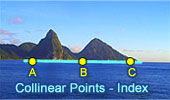# Collinear Points, Theorems and Problems - Table of Content 3Geometry Problem 1291.
Three Squares, Collinear Vertices, Midpoint, Congruence.

Geometry Problem 1280.
Quadrilateral, Perpendicular Diagonals, Isosceles Right Triangles, 45 Degrees, Collinear Points.

Geometry Problem 1255.
Right Triangle, Angle, 90 Degrees, Perpendicular, Collinear Points.

Geometry Problem 1242.
Quadrilateral, Four Interior and Exterior Angle Bisectors, Collinearity, Concurrent Lines. Mobile Apps.

Geometry Problem 1232
Triangle, Altitudes, Orthic Triangle, Collinearity, Orthic Axis.

Geometry Problem 1221
Intersecting Circles, Chord, Tangent, Parallel Chords, Collinear Points.

Geometry Problem 1189
Circle, Tangent Line, Secant, Chord, Collinear Points.

Geometry Problem 1176
Cyclic Quadrilateral, Diagonals, Six Diameters, Circles, Collinear Points, Concurrent Lines.

Geometry Problem 1169
Complete Quadrilateral, Orthocenters of the Component Triangles, Collinear Points, Ortholine, Steiner-line, Orthocentric line.

Geometry Problem 1167.
Triangle, Circle, Circumcircle, Perpendicular, 90 Degrees, Collinear Points.

Geometry Problem 1143.
Triangle, Circumcircle, Tangent Line, Reflection of a Point over a Line, Tangent Circles, Collinear Points.

Geometry Problem 896
Triangle, Orthocenter, Midpoint, Median, Intersecting Circles, Diameter, Collinear Points.
GeoGebra, HTML5 Animation for Tablets

Geometry Problem 895
Triangle, Incircle, Tangency Points, Parallel line, Collinear Points.
GeoGebra, HTML5 Animation for Tablets

Geometry Problem 893
Triangle, Circumcircle, Incenter, Circle, Tangent, Collinear Points.
GeoGebra, HTML5 Animation for Tablets

Geometry Problem 870
Triangle, Median, Three Squares, Centers, Vertices, Collinear Points, Midpoint.

Geometry Problem 869
Triangle, Median, Three Equilateral Triangles, Collinear Points, Midpoint.

Go to page:  Previous | 1 | 2 | 3 | 4 | 5 | 6 | 7 | 8 | 9 | 10 | Next